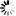# Publications in area "Random Graph Theory"

All || By Area || By Year

Title Published SHARCNET Authors Area
1. 2012 Pawel Pralat Random Graph Theory Mathematical Modeling & Simulation
2. 2010 R. Angelova, M. Lipczak, E. Milios, and P. Pralat Random Graph Theory Mathematical Modeling & Simulation
3. 2010 P.Gordinowicz and P.Pralat Random Graph Theory Mathematical Modeling & Simulation
4. 2010 S.Gaspers, M.E.Messinger, R.J.Nowakowski, and P.Pralat Random Graph Theory Mathematical Modeling & Simulation
5. 2008 Pawel Pralat Random Graph Theory Mathematical Modeling & Simulation
6. 2008 Pawel Pralat Random Graph Theory Mathematical Modeling & Simulation
7. 2008 R. Angelova, M. Lipczak, E. Milios, P. Pralat Random Graph Theory Mathematical Modeling & Simulation
8. 2007 Reza Dorrigiv, Alejandro Lopez-Ortiz, Pawel Pralat Random Graph Theory Mathematical Modeling & Simulation
Back to all areas